• 轮廓介绍
• 压缩轮廓
• 图像的矩
• 与轮廓有关的更多函数
• 过滤轮廓
• 识别轮廓
• 匹配轮廓

# 技术要求

• Python 和 OpenCV
• 特定于 Python 的 IDE
• NumPy 和 Matplotlib 包
• Git 客户端

# 轮廓介绍

def get_one_contour():
"""Returns a 'fixed' contour"""

cnts = [np.array(
[[[600, 320]], [[563, 460]], [[460, 562]], [[320, 600]], [[180, 563]], [[78, 460]], [[40, 320]], [[77, 180]], [[179, 78]], [[319, 40]], [[459, 77]], [[562, 179]]], dtype=np.int32)]
return cnts


contours = get_one_contour()
print("'detected' contours: '{}' ".format(len(contours)))
print("contour shape: '{}'".format(contours.shape))


[[[600 320]]
[[563 460]]
[[460 562]]
[[320 600]]
[[180 563]]
[[ 78 460]]
[[ 40 320]]
[[ 77 180]]
[[179 78]]
[[319 40]]
[[459 77]]
[[562 179]]]


squeeze = np.squeeze(cnt)


[[600 320]
[563 460]
[460 562]
[320 600]
[180 563]
[ 78 460]
[ 40 320]
[ 77 180]
[179 78]
[319 40]
[459 77]
[562 179]]


def draw_contour_points(img, cnts, color):
"""Draw all points from a list of contours"""

for cnt in cnts:
squeeze = np.squeeze(cnt)

for p in squeeze:
p = array_to_tuple(p)
cv2.circle(img, p, 10, color, -1)

return img


def array_to_tuple(arr):
"""Converts array to tuple"""

return tuple(arr.reshape(1, -1))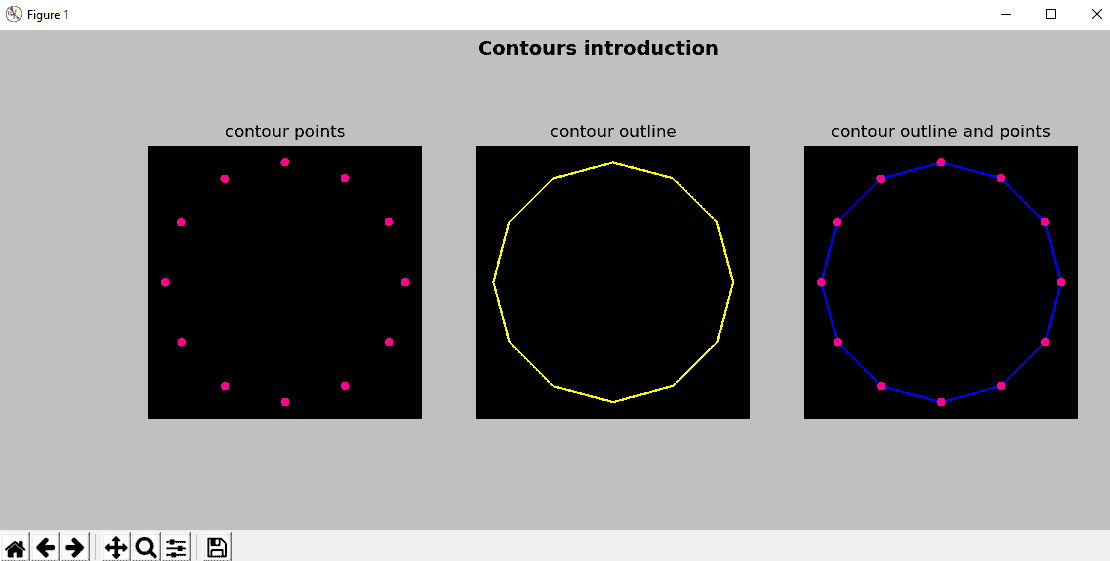def build_sample_image():
"""Builds a sample image with basic shapes"""

# Create a 500x500 gray image (70 intensity) with a rectangle and a circle inside:
img = np.ones((500, 500, 3), dtype="uint8") * 70
cv2.rectangle(img, (100, 100), (300, 300), (255, 0, 255), -1)
cv2.circle(img, (400, 400), 100, (255, 255, 0), -1)

return img


def build_sample_image_2():
"""Builds a sample image with basic shapes"""

# Create a 500x500 gray image (70 intensity) with a rectangle and a circle inside (with internal contours):
img = np.ones((500, 500, 3), dtype="uint8") * 70
cv2.rectangle(img, (100, 100), (300, 300), (255, 0, 255), -1)
cv2.rectangle(img, (150, 150), (250, 250), (70, 70, 70), -1)
cv2.circle(img, (400, 400), 100, (255, 255, 0), -1)
cv2.circle(img, (400, 400), 50, (70, 70, 70), -1)


contours_introduction_2.py中，在将图像加载之后，我们将其转换为灰度并设置了阈值以获得二进制图像。 此二进制图像稍后将用于使用cv2.findContours()函数查找轮廓。 如前所述，创建的图像仅具有圆形和正方形。 因此，调用cv2.findContours()将找到所有这些创建的轮廓。 cv2.findContours()方法的签名如下：

cv2.findContours(image, mode, method[, contours[, hierarchy[, offset]]]) -> image, contours, hierarchy


OpenCV 提供cv2.findContours()，可用于检测二进制图像（例如，阈值运算后生成的图像）​​中的轮廓。 该函数实现了中通过边界进行数字化二进制图像的拓扑结构分析中定义的算法。 应当注意，在 OpenCV 3.2 之前，源图像将已被修改，并且自 OpenCV 3.2 起，在调用此函数后将不再修改源图像。 源图像被视为二进制图像，其中非零像素被视为 1。 该函数返回检测到的轮廓，每个轮廓包含所有检索到的定义边界的点。

• hierarchy[i]：同一层级上的下一个轮廓的索引
• hierarchy[i]：在相同层次级别上的先前轮廓的索引
• hierarchy[i]：第一个子轮廓的索引
• hierarchy[i]：父轮廓的索引

hierarchy[i][j]中的负值表示没有下一个轮廓j=0，没有上一个轮廓j=1，没有子轮廓j=2或没有父轮廓j=3。 最后，method参数设置检索与每个检测到的轮廓有关的点时使用的近似方法。 下一部分将进一步说明此参数。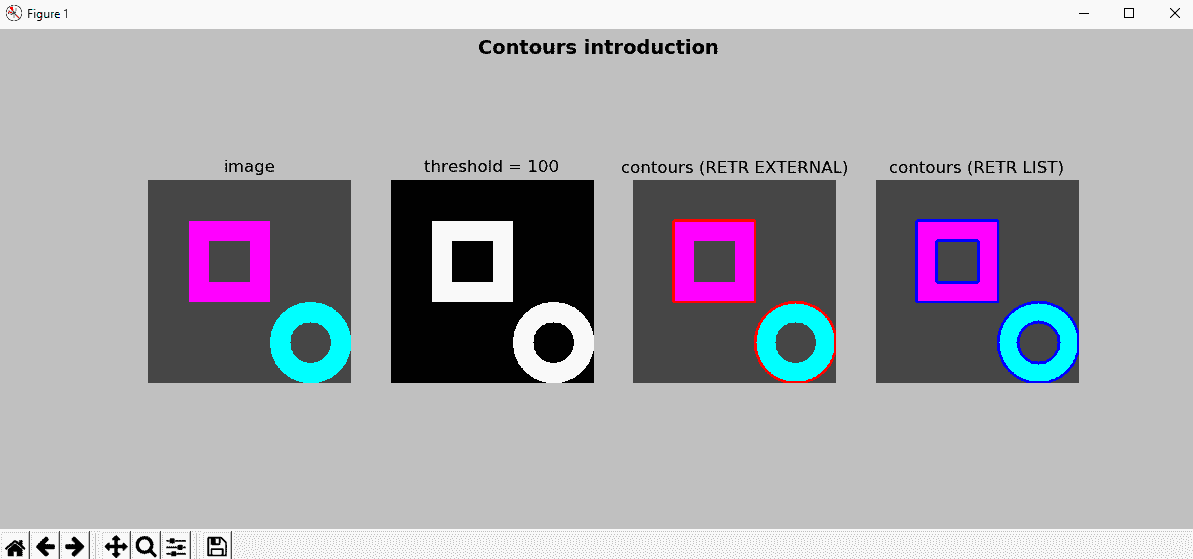# 压缩轮廓

cv2.CHAIN_APPROX_SIMPLE方法可用于压缩检测到的轮廓，因为它压缩轮廓的水平，垂直和对角线部分，仅保留端点。 例如，如果我们使用cv2.CHAIN_APPROX_SIMPLE压缩矩形的轮廓，则它将仅由四个点组成。

• 余弦度量
• K 曲率度量
• 一种曲率度量（2k = 1

contours_approximation_method.py中，用于method参数的上述四个标记（cv2.CHAIN_APPROX_NONEcv2.CHAIN_APPROX_SIMPLEcv2.CHAIN_APPROX_TC89_L1cv2.CHAIN_APPROX_TC89_KCOS）用于编码图像中的两个检测到的轮廓。 下一个屏幕截图中可以看到此脚本的输出：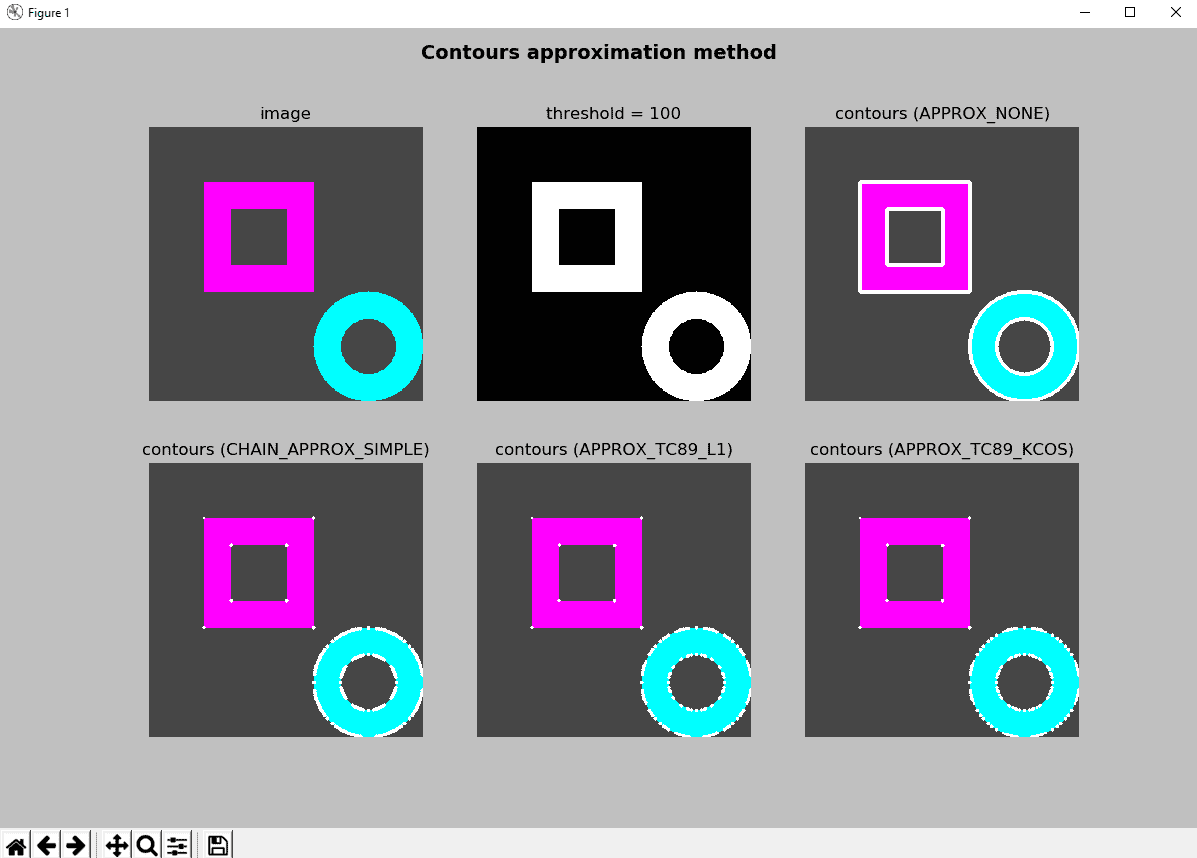# 图像的矩

cv2.moments()可用于计算直到向量形状或栅格化形状的三阶的所有矩。

retval = cv.moments(array[, binaryImage])


M = cv2.moments(contours)


{'m00': 235283.0, 'm10': 75282991.16666666, 'm01': 75279680.83333333, 'm20': 28496148988.333332, 'm11': 24089788592.25, 'm02': 28492341886.0, 'm30': 11939291123446.25, 'm21': 9118893653727.8, 'm12': 9117775940692.967, 'm03': 11936167227424.852, 'mu20': 4408013598.184406, 'mu11': 2712402.277420044, 'mu02': 4406324849.628765, 'mu30': 595042037.7265625, 'mu21': -292162222.4824219, 'mu12': -592577546.1586914, 'mu03': 294852334.5449219, 'nu20': 0.07962727021646843, 'nu11': 4.8997396280458296e-05, 'nu02': 0.07959676431294238, 'nu30': 2.2160077537124397e-05, 'nu21': -1.0880470778779139e-05, 'nu12': -2.2068296922023203e-05, 'nu03': 1.0980653771087236e-05}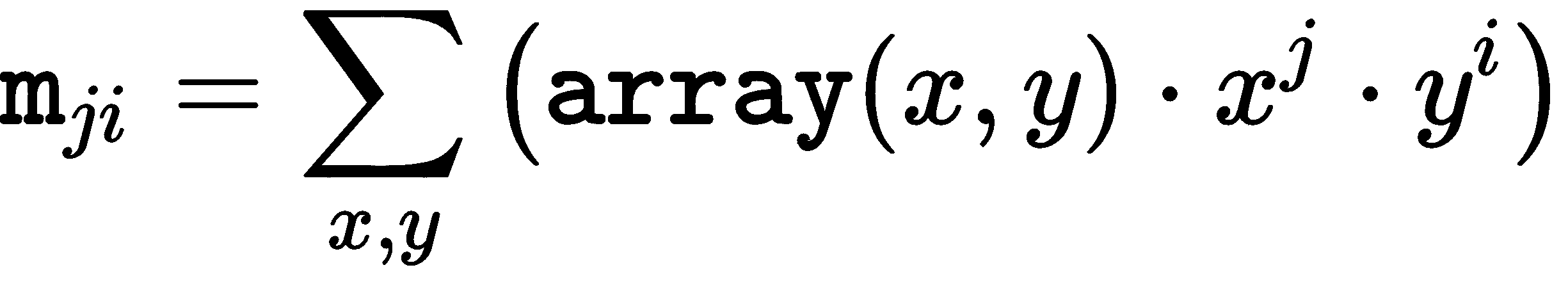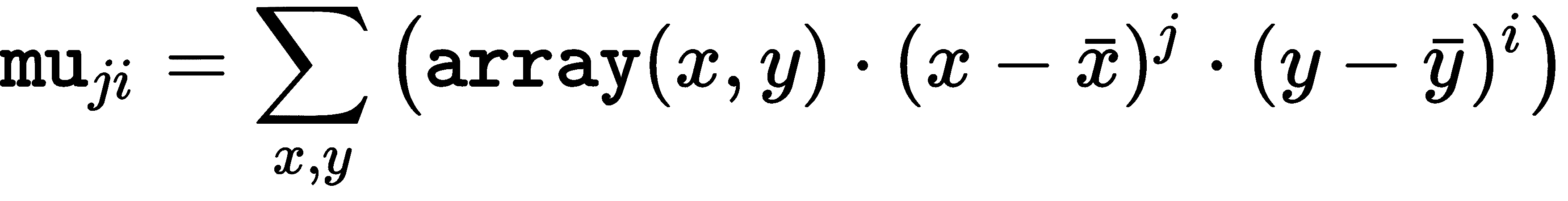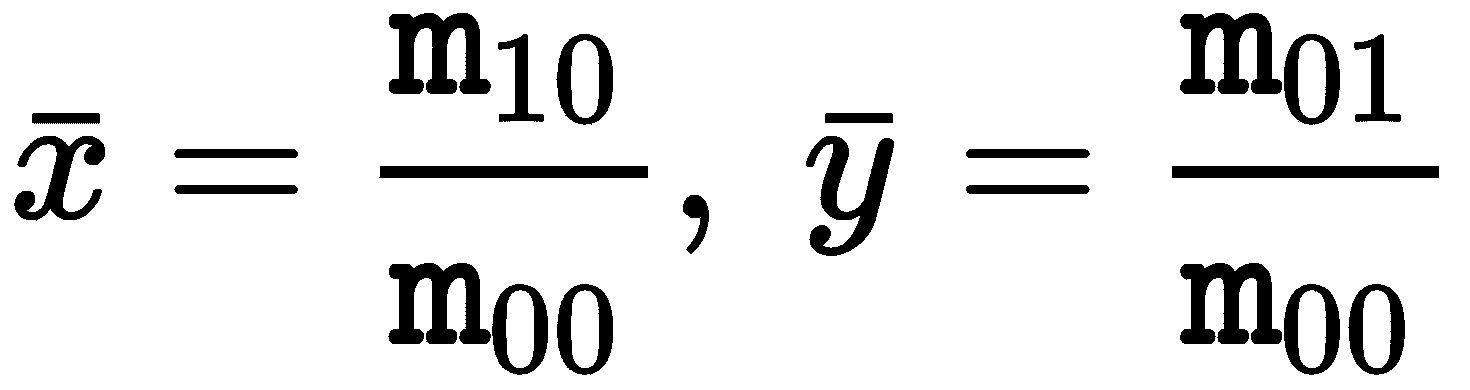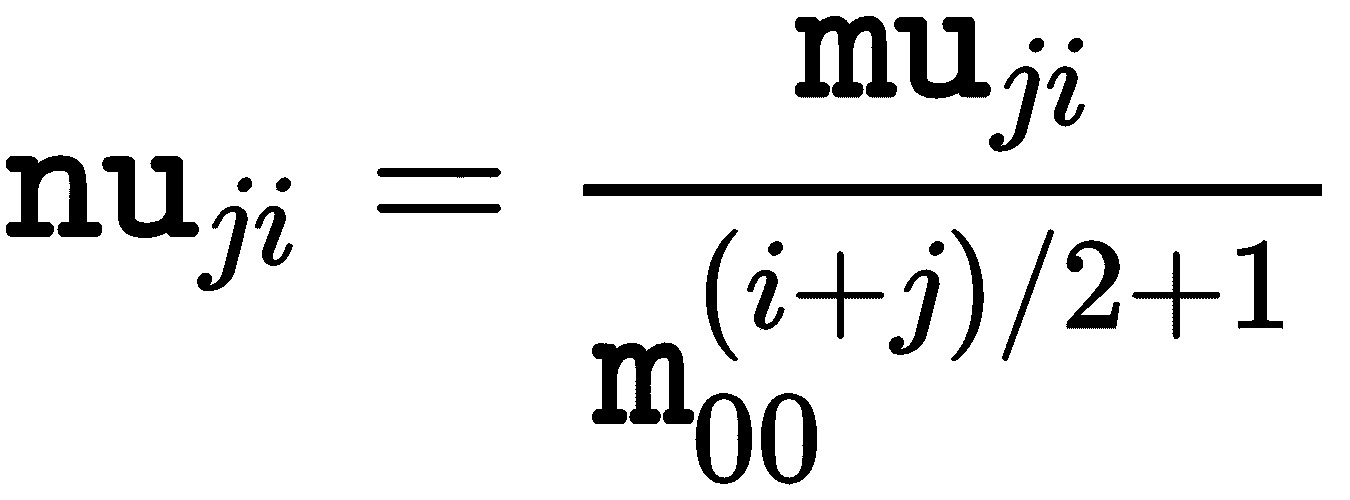mu = m, nu = 1, nu = mu = mu = mu = 0


# 一些基于矩的对象特征

contours_analysis.py中，我们将首先计算检测到的轮廓的矩，然后，将计算一些物体特征：

M = cv2.moments(contours)
print("Contour area: '{}'".format(cv2.contourArea(contours)))
print("Contour area: '{}'".format(M['m00']))


print("center X : '{}'".format(round(M['m10'] / M['m00'])))
print("center Y : '{}'".format(round(M['m01'] / M['m00'])))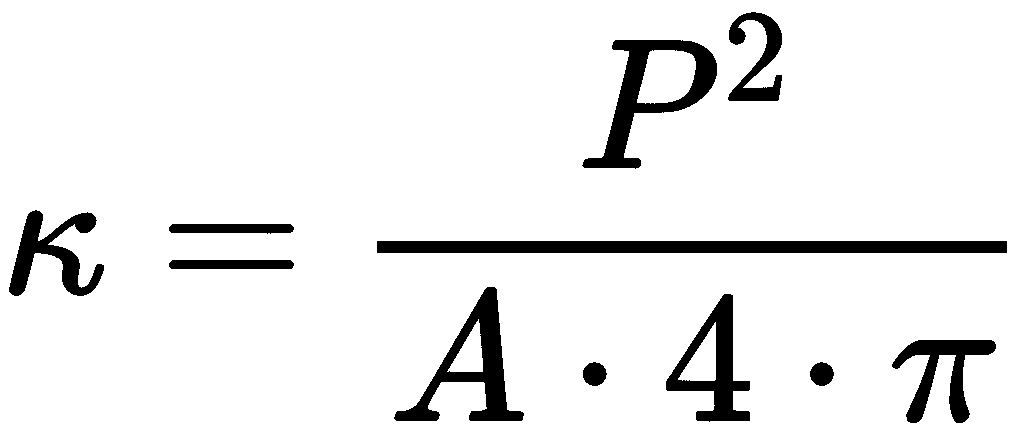P是轮廓的周长，A是相应的面积。 在正圆的情况下，结果为1； 获得的值越高，则圆形越小。

def roundness(contour, moments):
"""Calculates the roundness of a contour"""

length = cv2.arcLength(contour, True)
k = (length * length) / (moments['m00'] * 4 * np.pi)
return k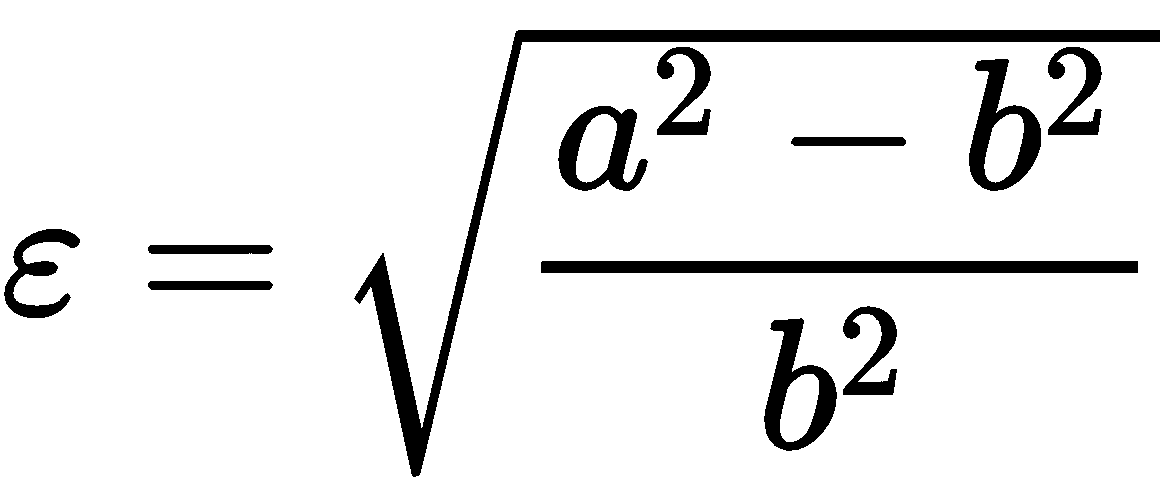def eccentricity_from_ellipse(contour):
"""Calculates the eccentricity fitting an ellipse from a contour"""

(x, y), (MA, ma), angle = cv2.fitEllipse(contour)

a = ma / 2
b = MA / 2

ecc = np.sqrt(a ** 2 - b ** 2) / a
return ecc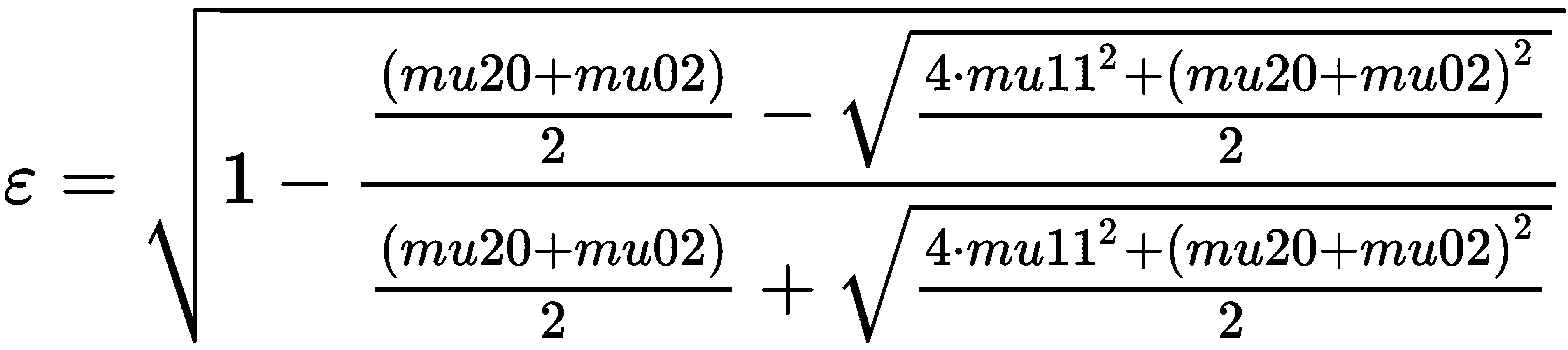def eccentricity_from_moments(moments):
"""Calculates the eccentricity from the moments of the contour"""

a1 = (moments['mu20'] + moments['mu02']) / 2
a2 = np.sqrt(4 * moments['mu11'] ** 2 + (moments['mu20'] - moments['mu02']) ** 2) / 2
ecc = np.sqrt(1 - (a1 - a2) / (a1 + a2))
return ecc


def aspect_ratio(contour):
"""Returns the aspect ratio of the contour based on the dimensions of the bounding rect"""

x, y, w, h = cv2.boundingRect(contour)
res = float(w) / h
return res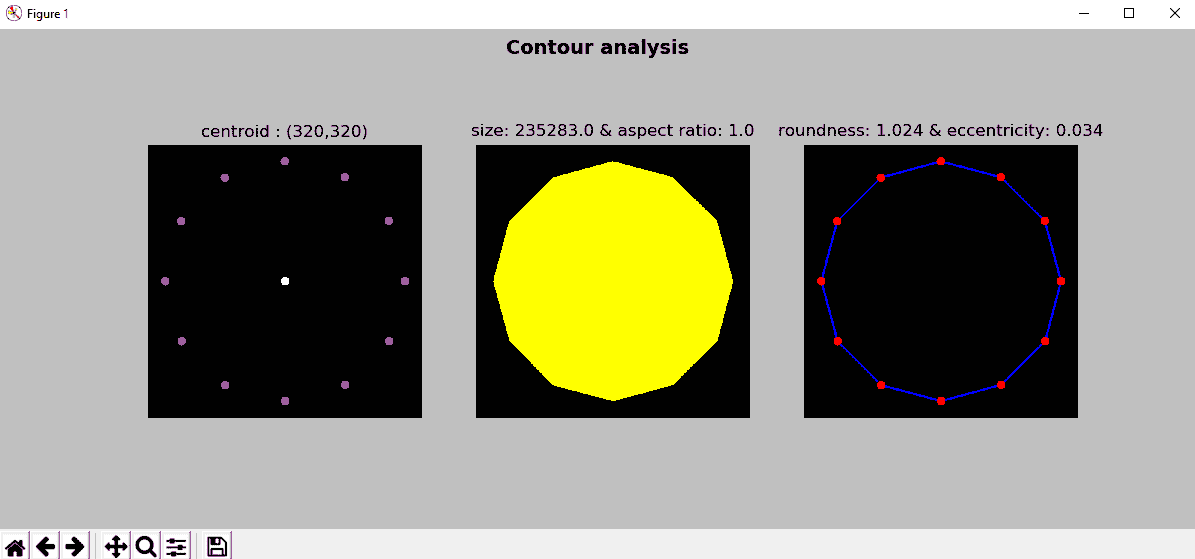def get_position_to_draw(text, point, font_face, font_scale, thickness):
"""Gives the coordinates to draw centered"""

text_size = cv2.getTextSize(text, font_face, font_scale, thickness)
text_x = point - text_size / 2
text_y = point + text_size / 2
return round(text_x), round(text_y)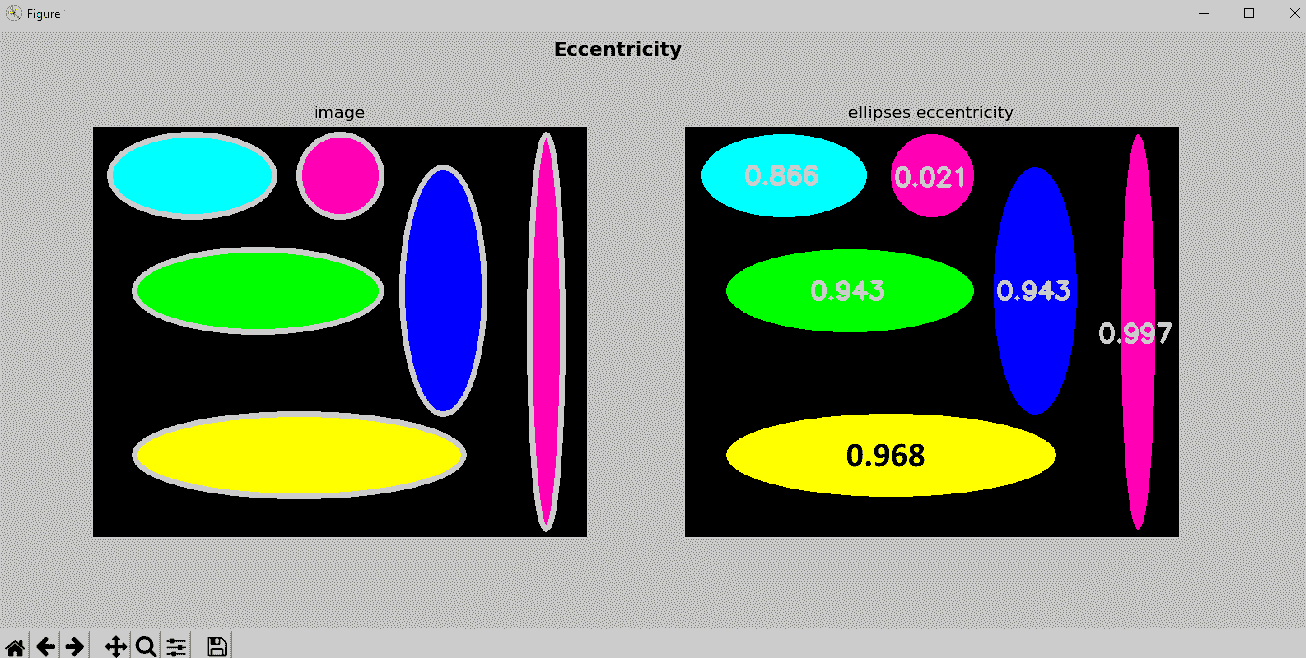# 胡矩不变量

cv2.HuMoments(m[, hu]) → hu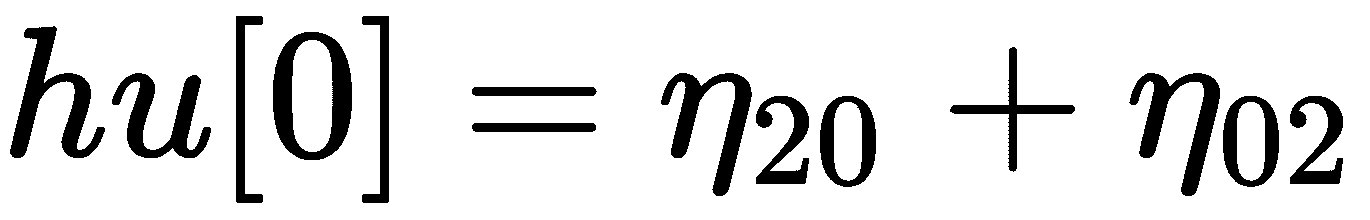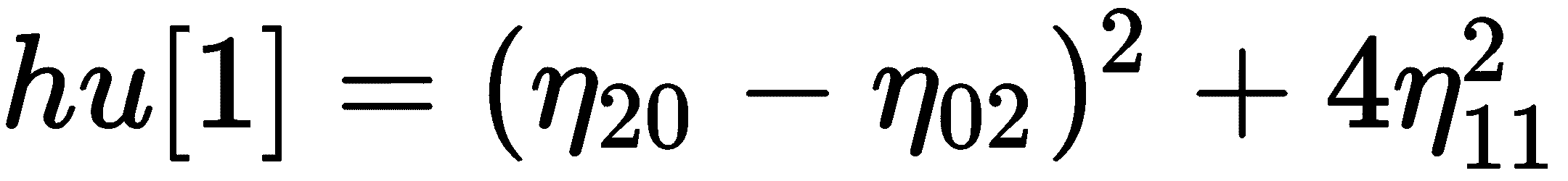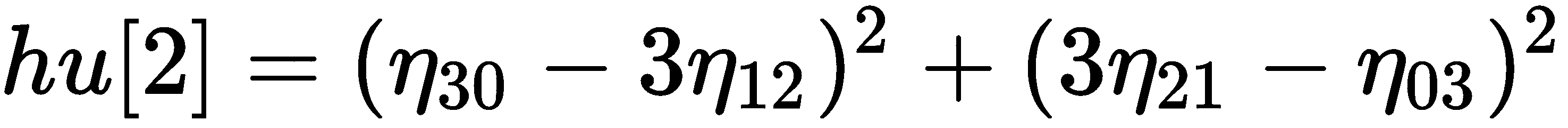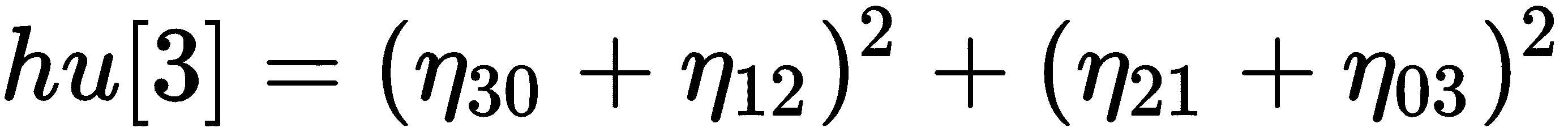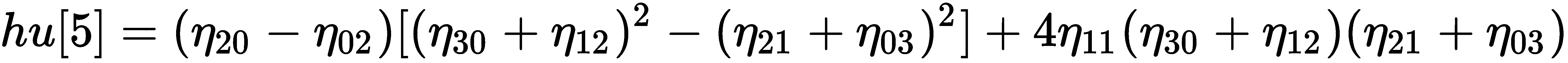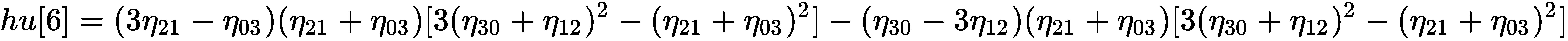η[ji]代表nu[ji]

contours_hu_moments.py脚本中，计算了七个胡矩不变量。 如前所述，我们必须首先使用cv2.moments()计算矩。 为了计算矩，该参数可以既是向量形状又是图像。 此外，如果binaryImage参数为true（仅用于图像），则输入图像中的所有非零像素将被视为 1。 在此脚本中，我们同时使用向量形状和图像来计算弯矩。 最后，利用计算出的矩，我们将计算出胡矩不变性。

# Load the image and convert it to grayscale:
gray_image = cv2.cvtColor(image, cv2.COLOR_BGR2GRAY)

# Apply cv2.threshold() to get a binary image
ret, thresh = cv2.threshold(gray_image, 70, 255, cv2.THRESH_BINARY)


# Compute moments:
M = cv2.moments(thresh, True)
print("moments: '{}'".format(M))

# Calculate the centroid of the contour based on moments:
x, y = centroid(M)

# Compute Hu moments:
HuM = cv2.HuMoments(M)
print("Hu moments: '{}'".format(HuM))


# Find contours
contours, hierarchy = cv2.findContours(thresh, cv2.RETR_EXTERNAL, cv2.CHAIN_APPROX_NONE)

# Compute moments:
M2 = cv2.moments(contours)
print("moments: '{}'".format(M2))

# Calculate the centroid of the contour based on moments:
x2, y2 = centroid(M2)

# Compute Hu moments:
HuM2 = cv2.HuMoments(M2)
print("Hu moments: '{}'".format(HuM2))


print("('x','y'): ('{}','{}')".format(x, y))
print("('x2','y2'): ('{}','{}')".format(x2, y2))


('x','y'): ('613','271')
('x2','y2'): ('613','270')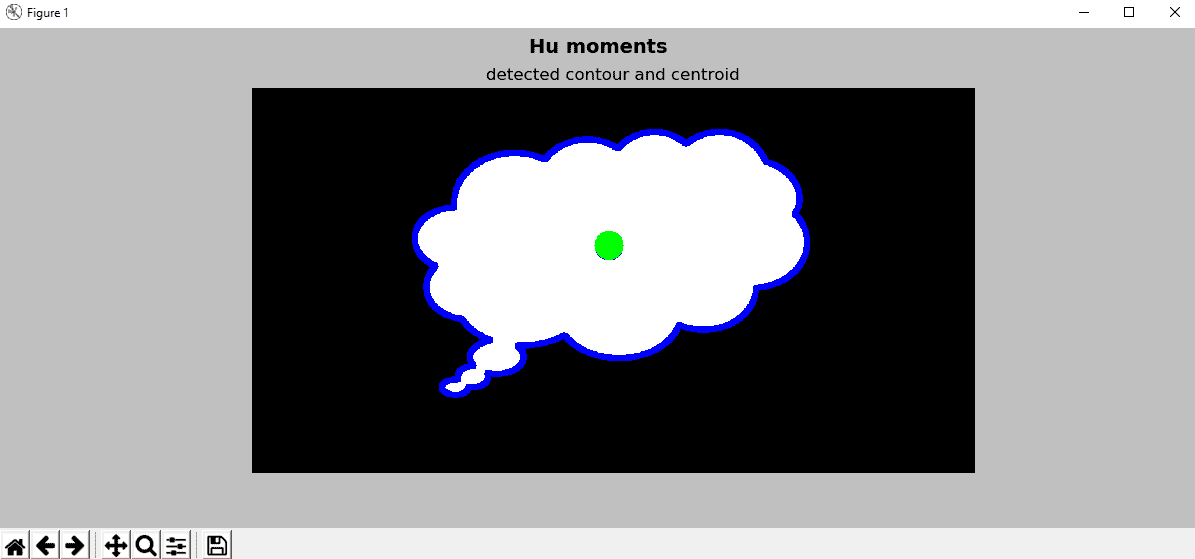contours_hu_moments_properties.py中，我们加载了三个图像。 第一个是原始的。 第二个与原件相对应，但旋转了 180 度。 第三个对应于原件的垂直反射。 这可以在脚本的输出中看到。 此外，我们打印从上述三个图像得出的计算得出的胡矩不变量。

# Load the images (cv2.imread()) and convert them to grayscale (cv2.cvtColor()):
gray_image_1 = cv2.cvtColor(image_1, cv2.COLOR_BGR2GRAY)
gray_image_2 = cv2.cvtColor(image_2, cv2.COLOR_BGR2GRAY)
gray_image_3 = cv2.cvtColor(image_3, cv2.COLOR_BGR2GRAY)

# Apply cv2.threshold() to get a binary image:
ret_1, thresh_1 = cv2.threshold(gray_image_1, 70, 255, cv2.THRESH_BINARY)
ret_2, thresh_2 = cv2.threshold(gray_image_2, 70, 255, cv2.THRESH_BINARY)
ret_2, thresh_3 = cv2.threshold(gray_image_3, 70, 255, cv2.THRESH_BINARY)

# Compute Hu moments cv2.HuMoments():
HuM_1 = cv2.HuMoments(cv2.moments(thresh_1, True)).flatten()
HuM_2 = cv2.HuMoments(cv2.moments(thresh_2, True)).flatten()
HuM_3 = cv2.HuMoments(cv2.moments(thresh_3, True)).flatten()

# Show calculated Hu moments for the three images:
print("Hu moments (original): '{}'".format(HuM_1))
print("Hu moments (rotation): '{}'".format(HuM_2))
print("Hu moments (reflection): '{}'".format(HuM_3))

# Plot the images:
show_img_with_matplotlib(image_1, "original", 1)
show_img_with_matplotlib(image_2, "rotation", 2)
show_img_with_matplotlib(image_3, "reflection", 3)

# Show the Figure:
plt.show()


Hu moments (original): '[ 1.92801772e-01 1.01173781e-02 5.70258405e-05 1.96536742e-06 2.46949980e-12 -1.88337981e-07 2.06595472e-11]'
Hu moments (rotation): '[ 1.92801772e-01 1.01173781e-02 5.70258405e-05 1.96536742e-06 2.46949980e-12 -1.88337981e-07 2.06595472e-11]'
Hu moments (reflection): '[ 1.92801772e-01 1.01173781e-02 5.70258405e-05 1.96536742e-06 2.46949980e-12 -1.88337981e-07 -2.06595472e-11]'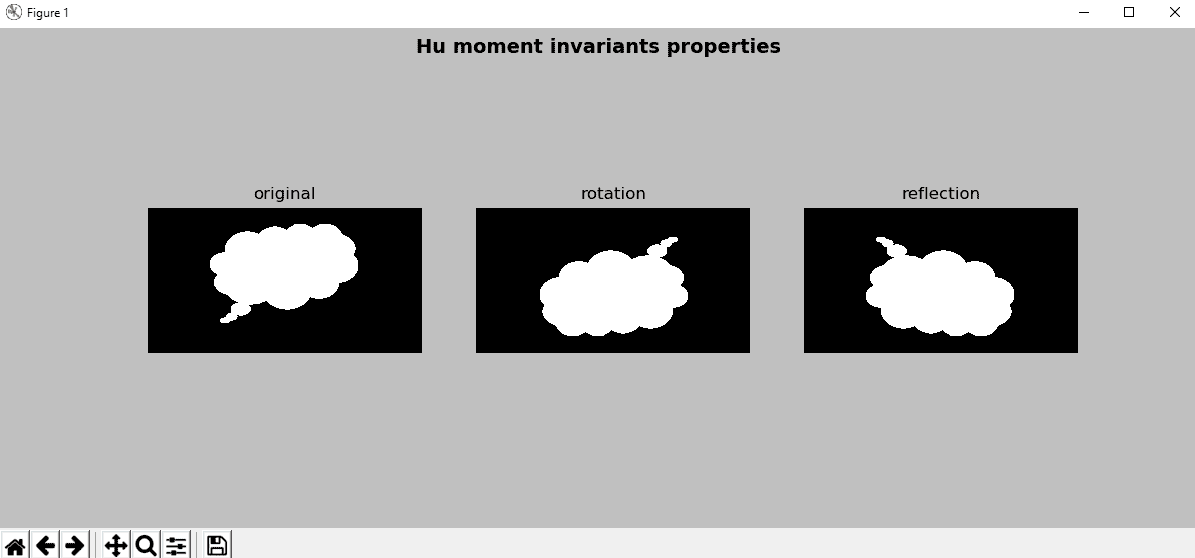# Zernike 矩

mahotas.features.zernike_moments(im, radius, degree=8, cm={center_of_mass(im)})


moments = mahotas.features.zernike_moments(image, 21)


# 与轮廓有关的更多函数

contours_functionality.py中，我们主要使用五个与轮廓相关的 OpenCV 函数和一个计算给定轮廓的极值的函数。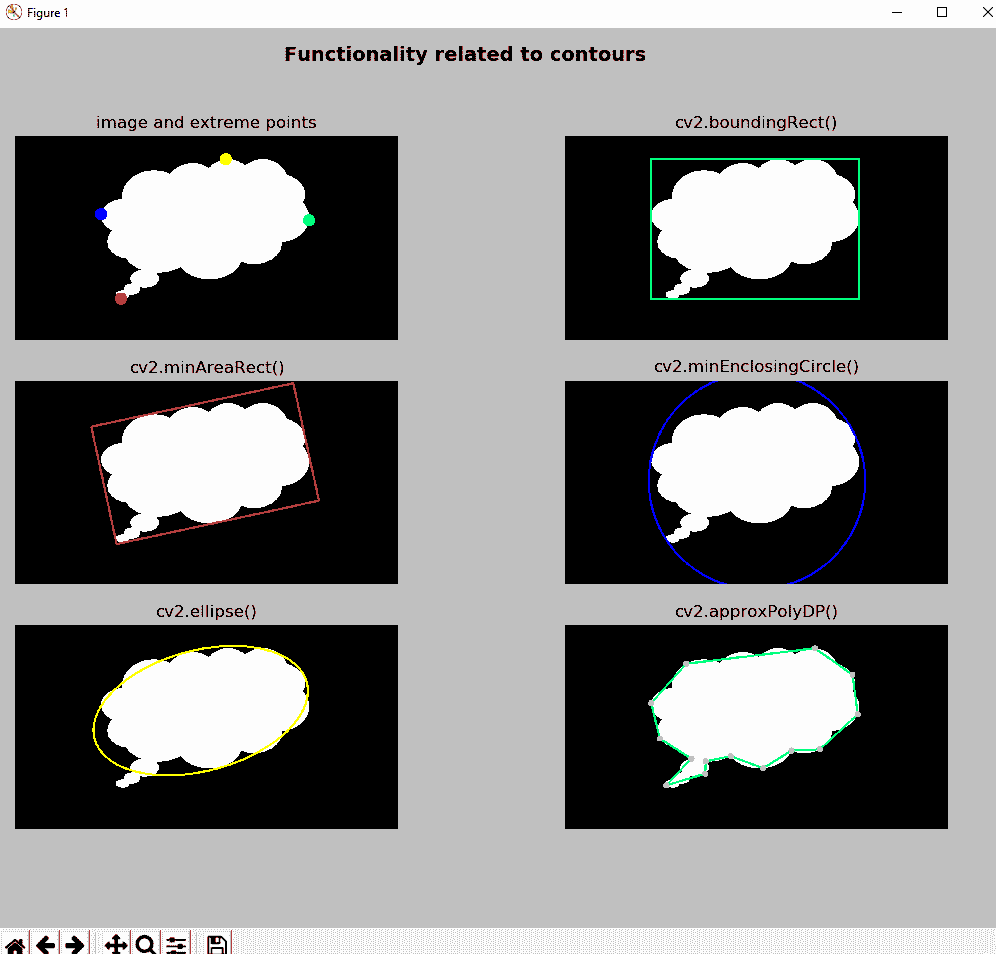cv2.boundingRect()返回包含轮廓的所有点的最小边界矩形：

x, y, w, h = cv2.boundingRect(contours)


cv2.minAreaRect()返回包含轮廓的所有点的最小旋转（如果需要）的矩形：

rotated_rect = cv2.minAreaRect(contours)


box = cv2.boxPoints(rotated_rect)


cv2.minEnclosingCircle()返回包含轮廓的所有点的最小圆（它返回中心和半径）：

(x, y), radius = cv2.minEnclosingCircle(contours)


cv2.fitEllipse()返回符合（具有最小的最小平方误差）轮廓的所有点的椭圆：

ellipse = cv2.fitEllipse(contours)


cv2.approxPolyDP()根据给定的精度返回给定轮廓的轮廓近似值。 此函数使用 Douglas-Peucker 算法。

epsilon参数确定精度，确定原始曲线与其近似之间的最大距离。 因此，所得轮廓是与给定轮廓相似的抽取轮廓，其点更少：

approx = cv2.approxPolyDP(contours, epsilon, True)


extreme_points()计算定义给定轮廓的四个极限点：

def extreme_points(contour):
"""Returns extreme points of the contour"""

index_min_x = contour[:, :, 0].argmin()
index_min_y = contour[:, :, 1].argmin()
index_max_x = contour[:, :, 0].argmax()
index_max_y = contour[:, :, 1].argmax()

extreme_left = tuple(contour[index_min_x])
extreme_right = tuple(contour[index_max_x])
extreme_top = tuple(contour[index_min_y])
extreme_bottom = tuple(contour[index_max_y])

return extreme_left, extreme_right, extreme_top, extreme_bottom


np.argmin()返回沿轴的最小值的索引。 在多次出现最小值的情况下，返回与第一次出现相对应的索引。 np.argmax()返回最大值的索引。 一旦计算出索引（例如index），我们将获得数组的相应组件（例如contour[index]-[[ 40 320]]），然后访问第一个组件（例如contour[index]-[ 40 320]）。 最后，我们将其转换为元组（例如tuple(contour[index])-(40,320)）。

index_min_x = contour[:, :, 0].argmin()
extreme_left = tuple(contour[index_min_x])


extreme_left = tuple(contour[contour[:, :, 0].argmin()])


# 过滤轮廓

def sort_contours_size(cnts):
""" Sort contours based on the size"""

cnts_sizes = [cv2.contourArea(contour) for contour in cnts]
(cnts_sizes, cnts) = zip(*sorted(zip(cnts_sizes, cnts)))
return cnts_sizes, cnts


coordinate = ['x', 'y', 'z']
value = [5, 4, 3]
result = zip(coordinate, value)
print(list(result))
c, v =  zip(*zip(coordinate, value))
print('c =', c)
print('v =', v)


[('x', 5), ('y', 4), ('z', 3)]
c = ('x', 'y', 'z')
v = (5, 4, 3)


coordinate = ['x', 'y', 'z']
value = [5, 4, 3]
print(sorted(zip(value, coordinate)))
c, v = zip(*sorted(zip(value, coordinate)))
print('c =', c)
print('v =', v)


[(3, 'z'), (4, 'y'), (5, 'x')]
c = (3, 4, 5)
v = ('z', 'y', 'x')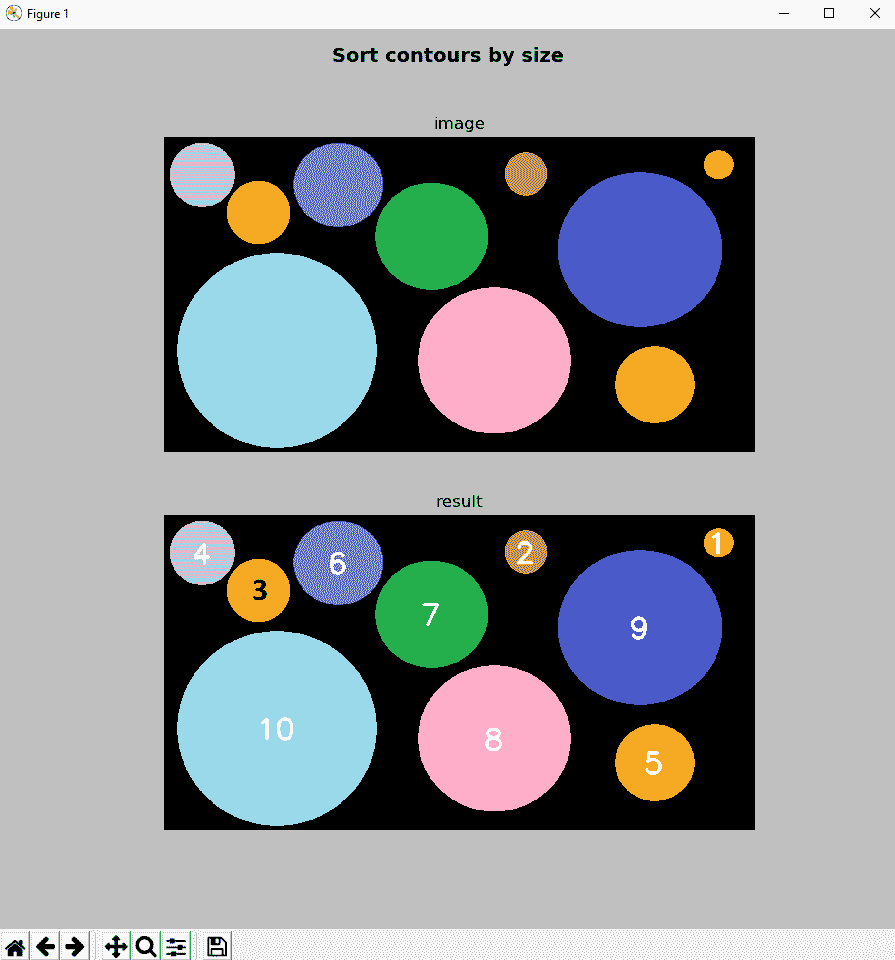# 识别轮廓

epsilon = 0.03 * perimeter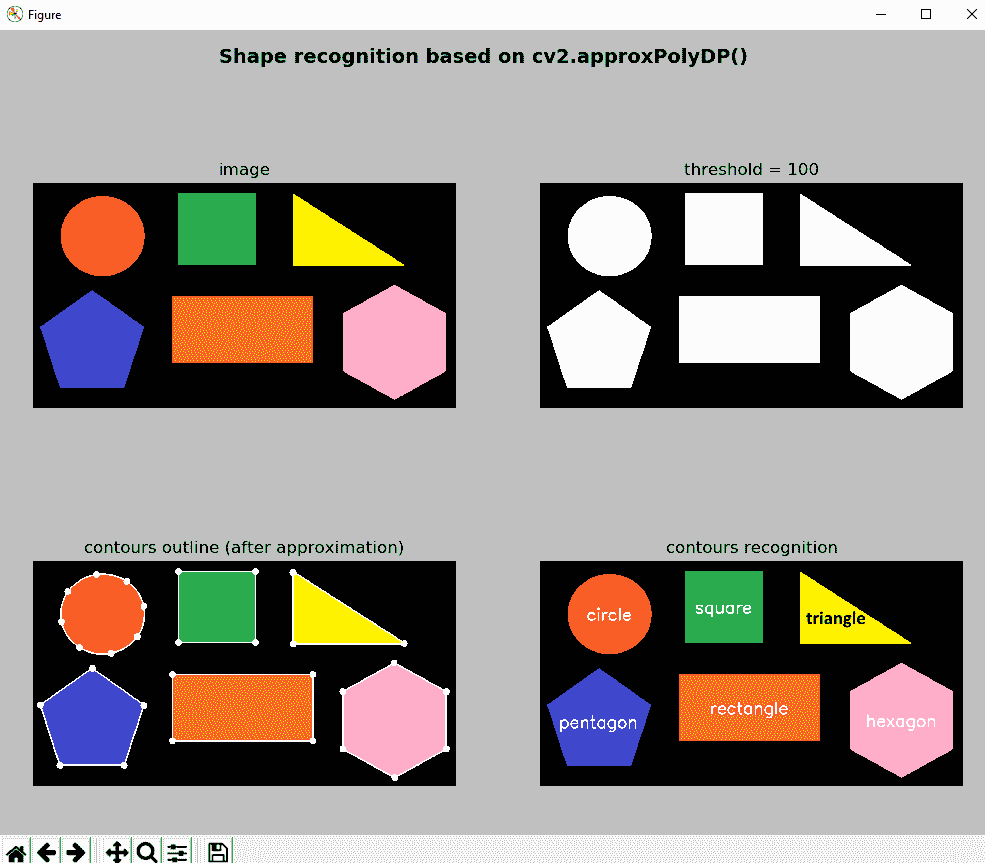# 匹配轮廓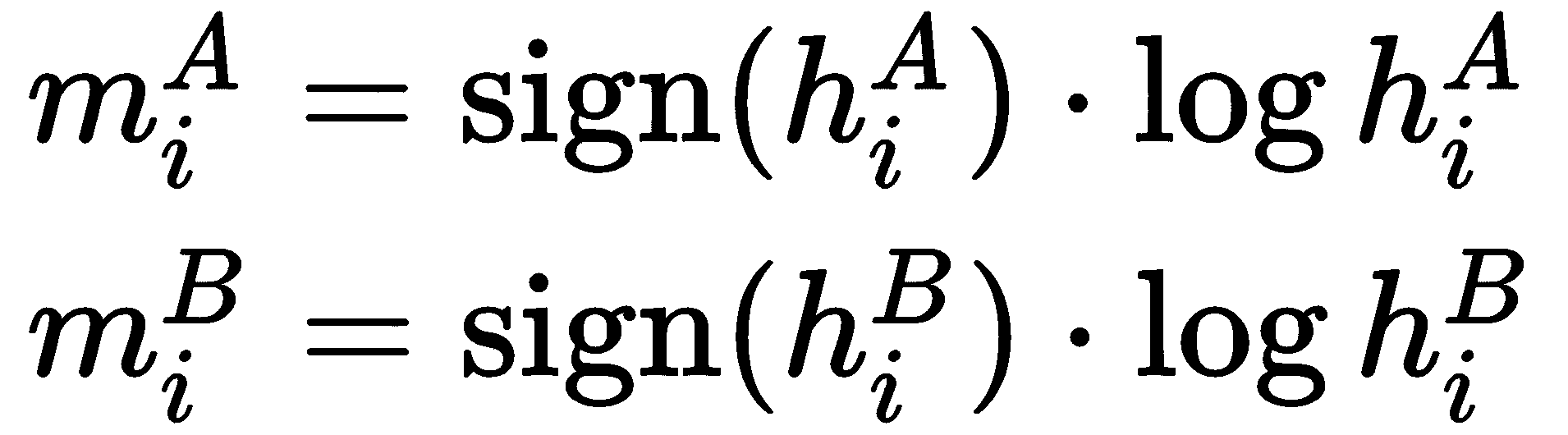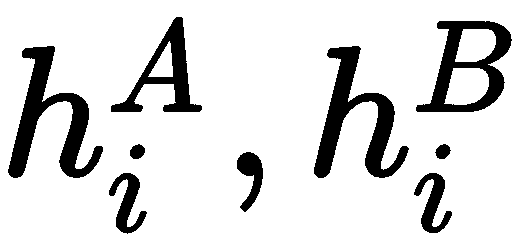分别是AB的胡矩。

• cv2.CONTOURS_MATCH_I1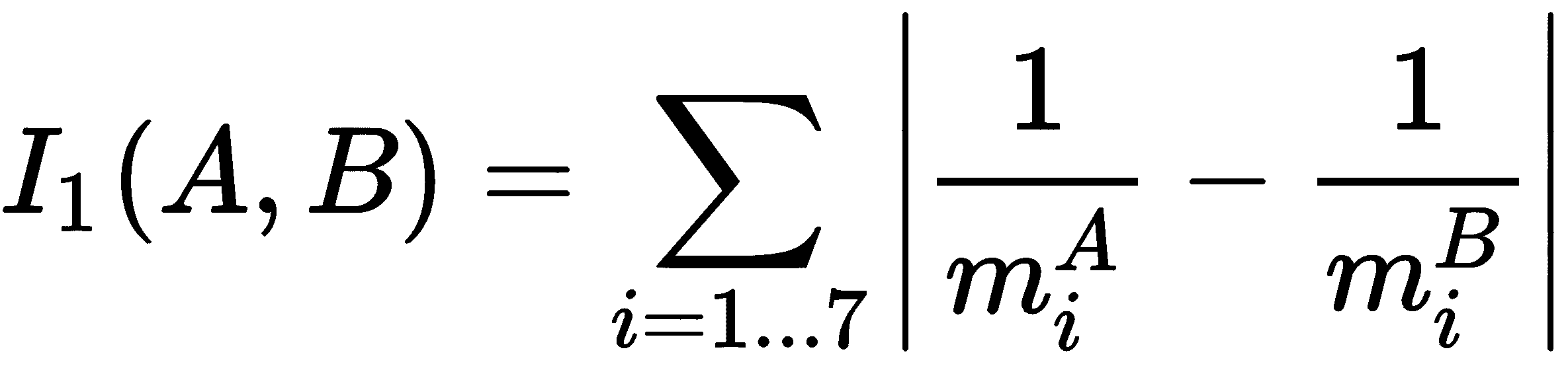• cv2.CONTOURS_MATCH_I2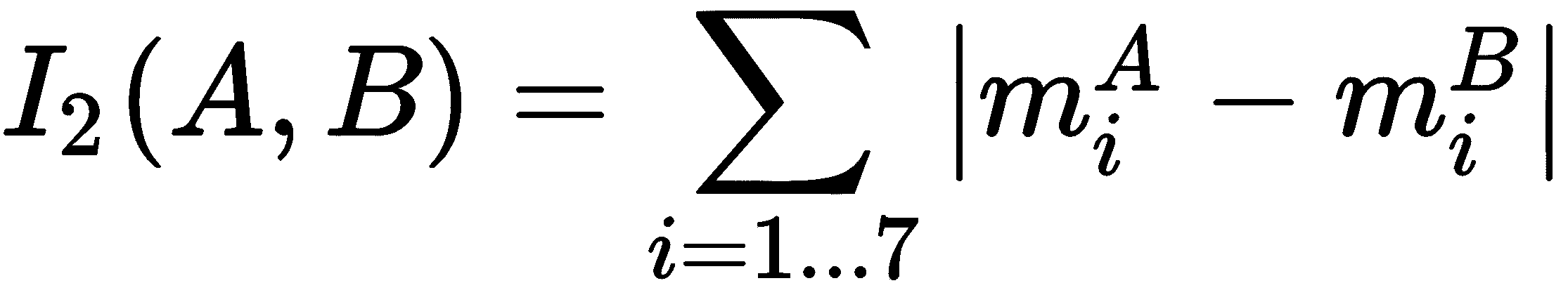• cv2.CONTOURS_MATCH_I3contours_matching.py中，我们利用cv2.matchShapes()将几个轮廓与一个完美的圆轮廓匹配。

1. 使用cv2.cvtColor()将其转换为灰度
2. 使用cv2.threshold()将其二值化
3. 使用cv2.findContours()查找轮廓

for contour in contours:
# Compute the moment of contour:
M = cv2.moments(contour)

# The center or centroid can be calculated as follows:
cX = int(M['m10'] / M['m00'])
cY = int(M['m01'] / M['m00'])

# We match each contour against the circle contour using the three matching modes:
ret_1 = cv2.matchShapes(contours_circle, contour, cv2.CONTOURS_MATCH_I1, 0.0)
ret_2 = cv2.matchShapes(contours_circle, contour, cv2.CONTOURS_MATCH_I2, 0.0)
ret_3 = cv2.matchShapes(contours_circle, contour, cv2.CONTOURS_MATCH_I3, 0.0)

# Get the positions to draw:
(x_1, y_1) = get_position_to_draw(str(round(ret_1, 3)), (cX, cY), cv2.FONT_HERSHEY_SIMPLEX, 1.2, 3)
(x_2, y_2) = get_position_to_draw(str(round(ret_2, 3)), (cX, cY), cv2.FONT_HERSHEY_SIMPLEX, 1.2, 3)
(x_3, y_3) = get_position_to_draw(str(round(ret_3, 3)), (cX, cY), cv2.FONT_HERSHEY_SIMPLEX, 1.2, 3)

# Write the obtainted scores in the result images:
cv2.putText(result_1, str(round(ret_1, 3)), (x_1, y_1), cv2.FONT_HERSHEY_SIMPLEX, 1.2, (255, 0, 0), 3)
cv2.putText(result_2, str(round(ret_2, 3)), (x_2, y_2), cv2.FONT_HERSHEY_SIMPLEX, 1.2, (0, 255, 0), 3)
cv2.putText(result_3, str(round(ret_3, 3)), (x_3, y_3), cv2.FONT_HERSHEY_SIMPLEX, 1.2, (0, 0, 255), 3)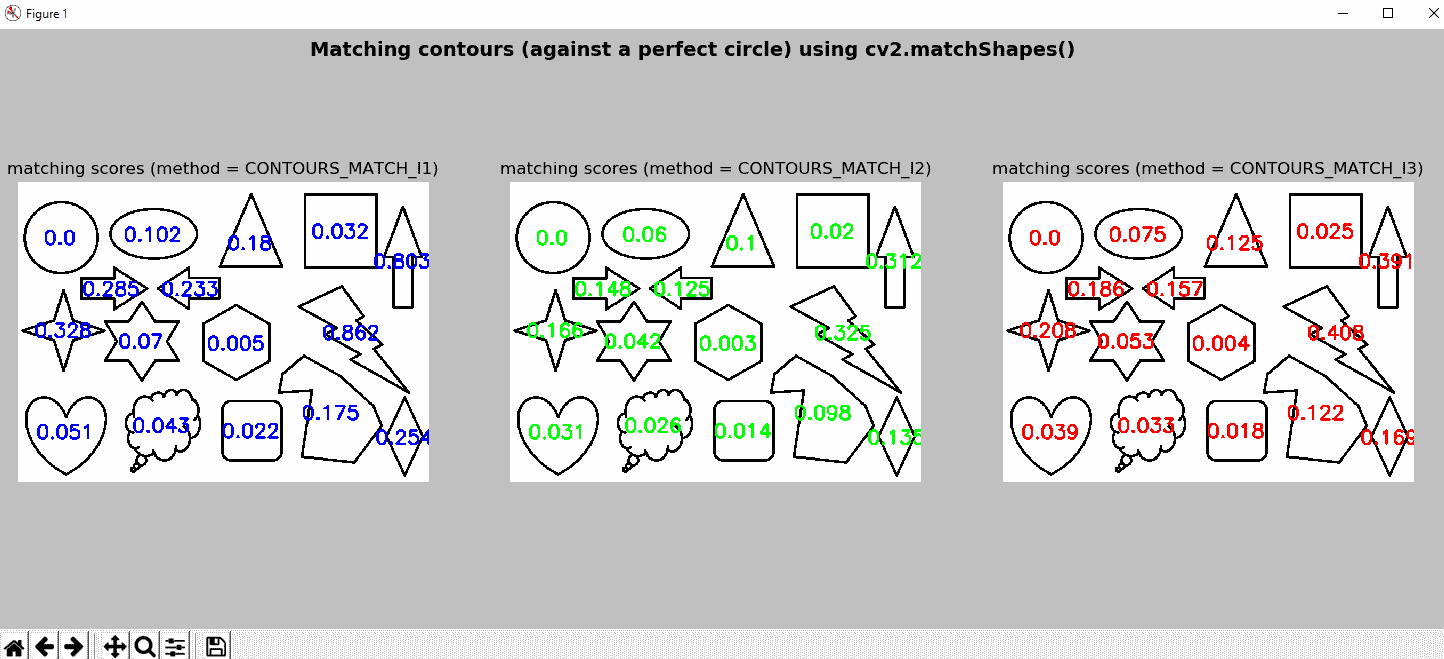# 问题

1. 如果要检测二进制图像中的轮廓，应该使用什么函数？
2. OpenCV 提供哪些四个标志来压缩轮廓？
3. OpenCV 提供什么函数来计算图像矩？
4. 什么矩提供轮廓的大小？
5. OpenCV 提供什么函数来计算七个胡矩不变量？
6. 如果要获得给定轮廓的轮廓近似值，应该使用什么函数？
7. 如本章所述，可以以更紧凑的方式覆盖contour_functionality.py脚本中定义的extreme_points()函数。 因此，请相应地覆盖它。
8. 如果要使用胡矩不变量作为特征来匹配轮廓，应该使用什么函数？